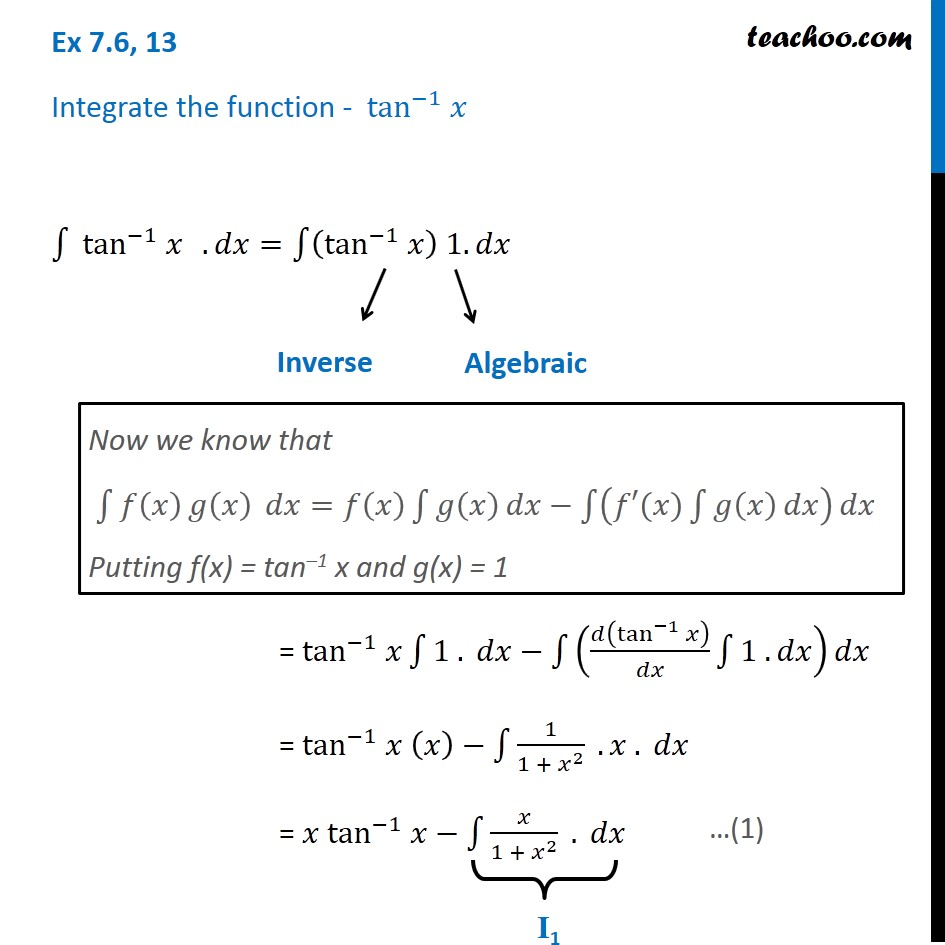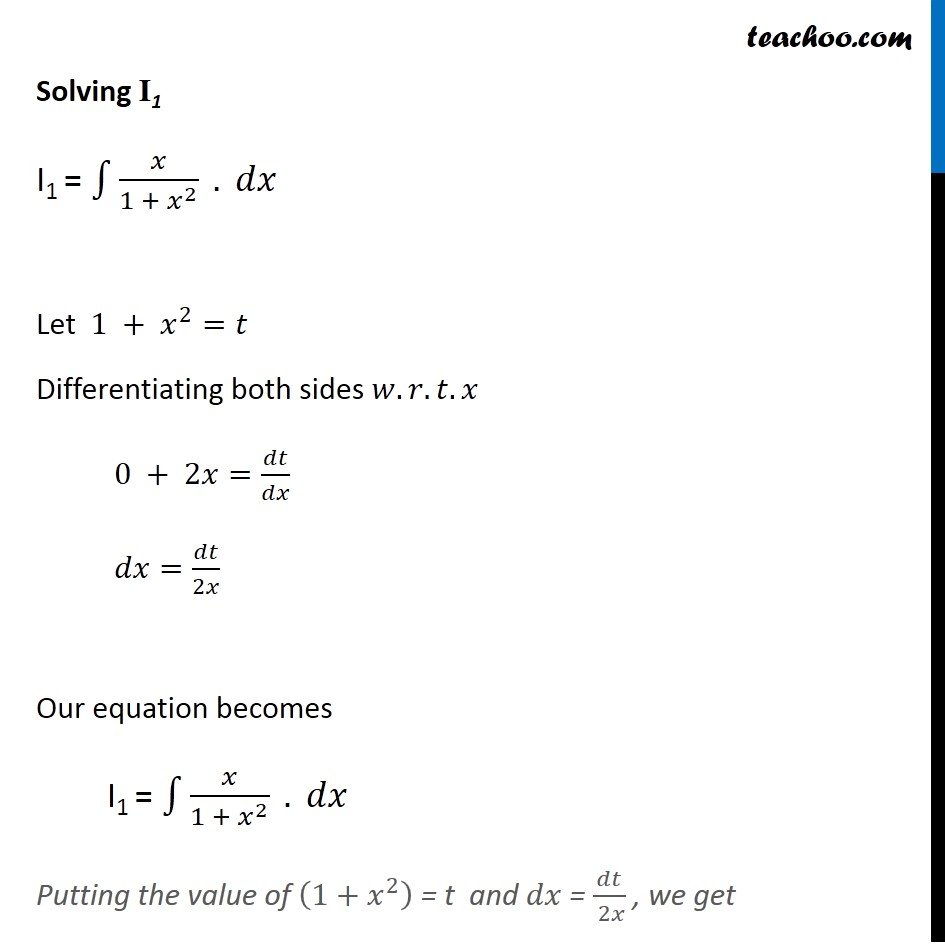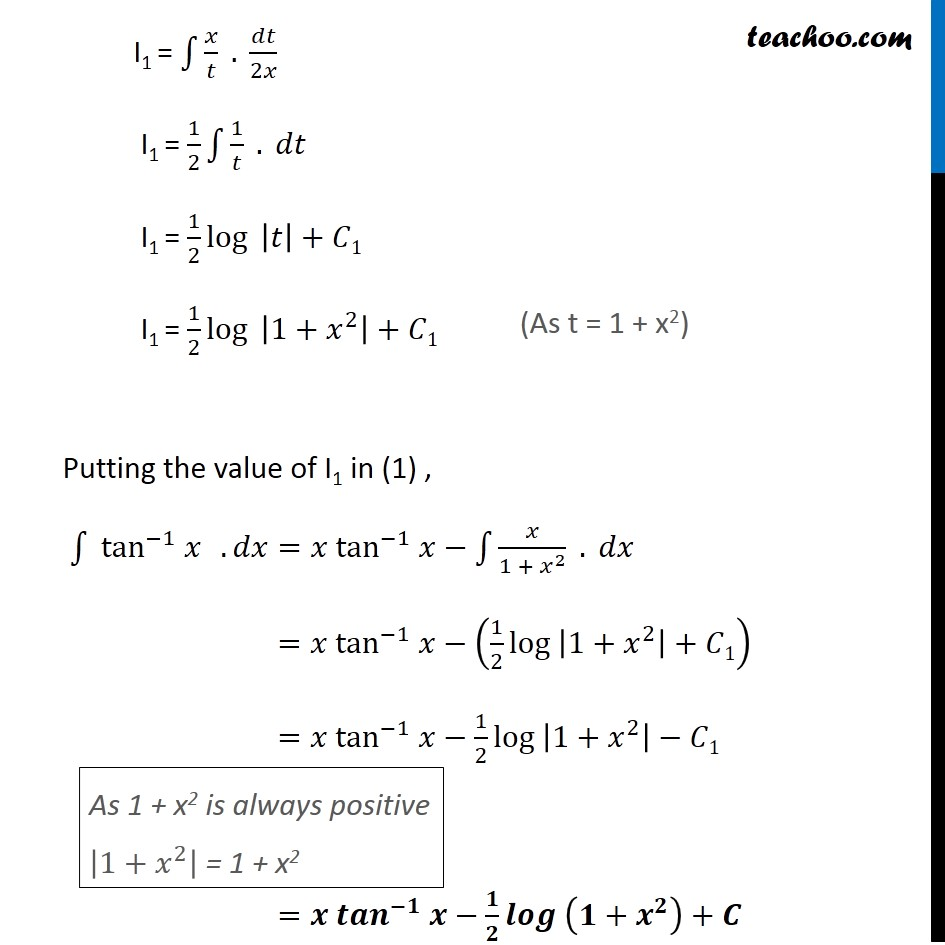Integration by parts

Chapter 7 Class 12 Integrals
Concept wiseLearn in your speed, with individual attention - Teachoo Maths 1-on-1 Class

### Transcript

Ex 7.6, 13 Integrate the function - tan^(−1) 𝑥 ∫1▒〖" " tan^(−1) 𝑥" " 〗 .𝑑𝑥=∫1▒〖(tan^(−1) 𝑥) 1.𝑑𝑥 " " 〗 = tan^(−1) 𝑥∫1▒〖1 .〗 𝑑𝑥−∫1▒(𝑑(tan^(−1)⁡𝑥 )/𝑑𝑥 ∫1▒〖1 .𝑑𝑥〗) 𝑑𝑥 = tan^(−1) 𝑥 (𝑥)−∫1▒1/(1 + 𝑥^2 ) . 𝑥 . 𝑑𝑥 = 𝑥 tan^(−1) 𝑥−∫1▒𝑥/(1 + 𝑥^2 ) . 𝑑𝑥 Now we know that ∫1▒〖𝑓(𝑥) 𝑔⁡(𝑥) 〗 𝑑𝑥=𝑓(𝑥) ∫1▒𝑔(𝑥) 𝑑𝑥−∫1▒(𝑓′(𝑥)∫1▒𝑔(𝑥) 𝑑𝑥) 𝑑𝑥 Putting f(x) = tan–1 x and g(x) = 1 Solving I1 I1 = ∫1▒𝑥/(1 + 𝑥^2 ) . 𝑑𝑥" " Let 1 + 𝑥^2=𝑡 Differentiating both sides 𝑤.𝑟.𝑡.𝑥 0 + 2𝑥=𝑑𝑡/𝑑𝑥 𝑑𝑥=𝑑𝑡/2𝑥 Our equation becomes I1 = ∫1▒𝑥/(1 + 𝑥^2 ) . 𝑑𝑥" " Putting the value of (1+𝑥^2 ) = t and 𝑑𝑥 = 𝑑𝑡/( 2𝑥) , we get I1 = ∫1▒𝑥/𝑡 . 𝑑𝑡/2𝑥 I1 = 1/2 ∫1▒1/𝑡 . 𝑑𝑡 I1 = 1/2 log⁡〖 |𝑡|〗+𝐶1 I1 = 1/2 log⁡〖 |1+𝑥^2 |〗+𝐶1 Putting the value of I1 in (1) , ∫1▒〖" " tan^(−1) 𝑥" " 〗 .𝑑𝑥=𝑥 tan^(−1) 𝑥−∫1▒𝑥/(1 + 𝑥^2 ) . 𝑑𝑥 =𝑥 tan^(−1) 𝑥−(1/2 〖log 〗⁡|1+𝑥^2 |+𝐶1) =𝑥 tan^(−1) 𝑥−1/2 〖log 〗⁡|1+𝑥^2 |−𝐶1 =𝒙 〖𝒕𝒂𝒏〗^(−𝟏) 𝒙−𝟏/𝟐 〖𝒍𝒐𝒈 〗⁡(𝟏+𝒙^𝟐 )+𝑪